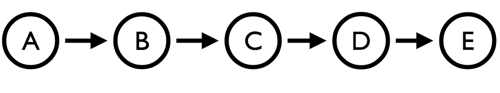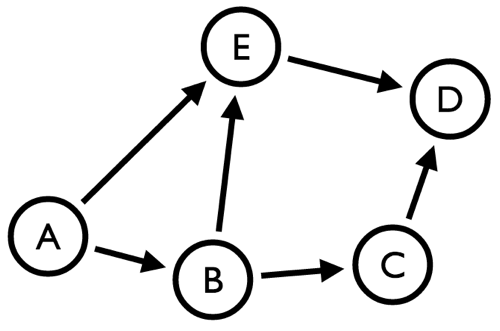# Graph Processing: Calculating betweenness centrality for an undirected graph using graphstream

Since I now spend most of my time surrounded by graphs I thought it'd be interesting to learn a bit more about graph processing, a topic my colleague Jim wrote about a couple of years ago.

I like to think of the types of queries you'd do with a graph processing engine as being similar in style graph global queries where you take most of the nodes in a graph into account and do some sort of calculation.

One of the interesting graph global algorithms that I've come across recently is 'betweenness centrality' which allows us to work out the centrality of each node with respect to the rest of the network/graph.

[Betweenness centrality] is equal to the number of shortest paths from all vertices to all others that pass through that node. Betweenness centrality is a more useful measure (than just connectivity) of both the load and importance of a node. The former is more global to the network, whereas the latter is only a local effect.

I wanted to find a library that I could play around with to get a better understanding of how this algorithm works and I came across graphstream which seems to do the job.

I was able to get up and running by creating a Maven pom file with the following dependencies:

``````
<project xmlns="http://maven.apache.org/POM/4.0.0" xmlns:xsi="http://www.w3.org/2001/XMLSchema-instance" xsi:schemaLocation="http://maven.apache.org/POM/4.0.0 http://maven.apache.org/xsd/maven-4.0.0.xsd">
<modelVersion>4.0.0</modelVersion>
<packaging>jar</packaging>
<artifactId>my-gs-project</artifactId>
<groupId>org.graphstream</groupId>
<version>1.0-SNAPSHOT</version>
<name>my-gs-project</name>
<description/>
<dependencies>
<dependency>
<groupId>org.graphstream</groupId>
<artifactId>gs-core</artifactId>
<version>1.0</version>
</dependency>
<dependency>
<groupId>org.graphstream</groupId>
<artifactId>gs-algo</artifactId>
<version>1.0</version>
</dependency>
</dependencies>
</project>

``````

I wanted to find a worked example which I could use to understand how betweenness centrality is calculated and I found one on slide 14 of these lecture notes from The University of Nevada.

The example graph looks like this:And to work out the betweenness centrality we need to take every pair of nodes and see which (if any) nodes a path between the two must pass through.

``````
A -> B: None
A -> C: B
A -> D: B, C
A -> E: B, C, D
B -> C: None
B -> D: C
B -> E: C, D
C -> D: None
C -> E: D
D -> E: None
``````

So for this example we end up with the following centrality values for each node:~~~ ~~~text A: 0 B: 3 C: 4 D: 3 E: 0 ~~~

If we run this through graph stream we'll see values double to this because it ignores the direction of a relationship (e.g. it's possible to go from B->A even though that relationship is actually only from A->B.):

``````
public class Spike {
public static void main(String[] args) {
Graph graph = new SingleGraph("Tutorial 1");

BetweennessCentrality bcb = new BetweennessCentrality();
bcb.init(graph);
bcb.compute();

System.out.println("A="+ A.getAttribute("Cb"));
System.out.println("B="+ B.getAttribute("Cb"));
System.out.println("C="+ C.getAttribute("Cb"));
System.out.println("D="+ D.getAttribute("Cb"));
System.out.println("E="+ E.getAttribute("Cb"));
}
}
``````
``````
A=0.0
B=6.0
C=8.0
D=6.0
E=0.0
``````

What we can learn from this calculation is that in this graph node 'C' is the most influential one because most paths between other nodes have to pass through it. There's not much in it though as nodes 'B' and 'D' are close behind.

Now that I had a better understanding of how to manually execute the algorithm I thought I should try and work out what the example from the documentation would return.

The example graph looks like this:And the paths between the nodes would be as follows:

Since I know graphstream treats the graph as being undirected I don't respect the direction of relationships in this calculation)

``````
A -> B: Direct Path Exists
A -> C: B
A -> D: E
A -> E: Direct Path Exists
B -> A: Direct Path Exists
B -> C: Direct Path Exists
B -> D: E or C
B -> E: Direct Path Exists
C -> A: B
C -> B: Direct Path Exists
C -> D: Direct Path Exists
C -> E: D or B
D -> A: E
D -> B: C or E
D -> C: Direct Path Exists
D -> E: Direct Path Exists
E -> A: Direct Path Exists
E -> B: Direct Path Exists
E -> C: D or B
E -> D: Direct Path Exists
``````

For some of these there were two potential paths so we give 1/2 a point to each node which leads to these totals

``````
A: 0
B: 3
C: 1
D: 1
E: 3
``````

If we run that through graphstream we'd expect to see the same values:

``````
public class Spike {
public static void main(String[] args) {
Graph graph = new SingleGraph("Tutorial 1");

BetweennessCentrality bcb = new BetweennessCentrality();
bcb.init(graph);
bcb.compute();

System.out.println("A="+ A.getAttribute("Cb"));
System.out.println("B="+ B.getAttribute("Cb"));
System.out.println("C="+ C.getAttribute("Cb"));
System.out.println("D="+ D.getAttribute("Cb"));
System.out.println("E="+ E.getAttribute("Cb"));
}
}
``````
``````
A=0.0
B=3.0
C=1.0
D=1.0
E=3.0
``````

This library does the job for some definition of betweenness centrality but ideally I'd like to have the direction of relationships taken into account so I'm going to give it a try with one of the other libraries that I've come across.

So far the other graph processing libraries I know of are graphchi, JUNG, Green-Marl and giraph but if you know of any others that I should try out please let me know.

** Update ** (27th July 2013)

While writing another post about betweenness centrality I realised I'd made a mistake in the calculations on this post so I've corrected those now.